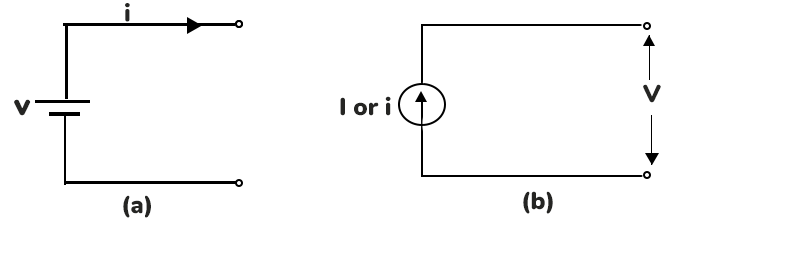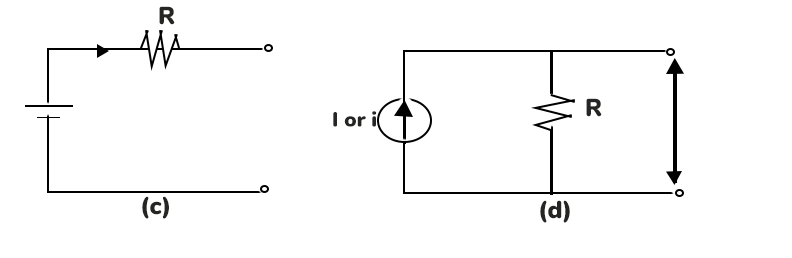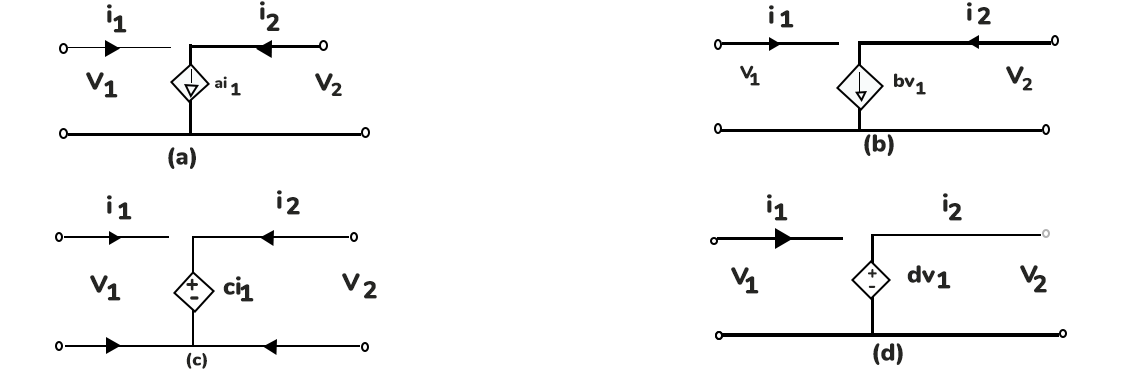### Independent Sources

• The ideal voltage source and current source are shown in figure 1.2• Fig. 1.2
1. ideal DC voltage source

2. ideal DC current source

3. practical voltage source

4. practical current source

• A voltage source is said to be idle if the output terminals are open such that i=0. A current source is said to be idle when the output terminals are shorted together with the voltage v=0. A non-ideal voltage source has series resistance and a non-ideal current source has parallel resistance.

Dependent or Controlled Sources:

• The ideal voltage source and current source are shown in figure 1.3. The voltage or current source whose value is not fixed but is dependent on a voltage or current at some other location in the network is known as dependent or controlled sources. There are four basic types of controlled sources.

• Fig.

1. Voltage Controlled Voltage Source (VCVS)

2. Voltage Controlled Current Source (VCVS)

3. Current Controlled Voltage Source (CCVS)

4. Current Controlled Current Source (CCCS)Ohms Law

• George Simon Ohm (1789 - 1854), found the relationship between voltage and current as expressed below.

and              (6)

• Where V is the voltage in volts (V), I is the current in Amperes (A), R is the resistance in ohms (Ω) and G is the conductance in Siemens (S).